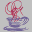[Previous] [Next] [Up] [Top]

# Assignment 5

Multivariate Data Analysis
Psychology 6140

The readings below are for the next two weeks. They bridge between material on eigenvectors and an introduction to ideas of regression
1. Green & Carroll,
• Chapter 5 (eigenvalues): This is a fairly long chapter, with a good deal of material rather more difficult than I'd like. Focus on the following sections (& see Stevens, for a brief summary): 5.1-5.2.2, 5.2.5-5.4.1, 5.5-5.6.4.
• Chapter 6 (Regression): 6.1-6.3; 6.6 -end
2. Tabachnick & Fidell (Regression), 5.1-5.4
3. Stevens, (eigenvalues) 2.6; (regression) 3.1-3.9

## Supplements

R: See the matlib vignettes, "eigen-ex1" and "eigen-ex2"
These demonstrate some properties of eigenvalues and eigenvectors.EigenExplorer
Some Java applets that allow you to visually explore the properties of eigenvectors and eigenvalues of 2x2 and 3x3 matrices. Go to Eigenvalues and Eigenvectors.

## Problems (due: next week)

From the Matrix Algebra Problems (MAP) handout: Try using R (or SAS/IML) to do the following problems:
1. Use the eigen() function to find the eigenvalues and eigenvectors of the following matrices:
```       +-     -+           +-        -+
A = | 5   4 |           |  2  2 -4 |
| 4   8 |     B =   |  2  4  2 |
+-     -+           | -4  2  2 |
+-        -+
```
e.g., r <- eigen(A).
2. Verify that for each matrix above, (a) the trace of the matrix is the sum of the latent roots, and (b) the determinant is the product.
3. Without direct calculation, find the latent roots of (a) A -1; (b) B 2.